• 可以录入学生的平时成绩和考试成绩，并统计学生的总成绩（计算方法：总成绩=考试成绩*80%+平时成绩20%）。按照总成绩学生进行排序，查询某个学生成绩（按照学号、姓名、成绩等关键词进行查询）。制定学生信息...
• C语言课程设计报告 学生成绩统计系统/C语言课程设计书设计题目： 学生成绩统计系统课程名称： C语言课程设计学 院 ：物理与光电工程学院专业班级： 电子科学与技术(1)班学 号 ：姓 名 ： 何灿豪联系方式 ：任课教师 ...

C语言课程设计报告 学生成绩统计系统

/

C语言课程设计书

设计题目： 学生成绩统计系统

课程名称： C语言课程设计

学 院 ：物理与光电工程学院

专业班级： 电子科学与技术(1)班

学 号 ：

姓 名 ： 何灿豪

联系方式 ：

任课教师 ： 刘力斌

2013 年 11月 10日

目录

设计目的 ··················· 2

任务要求 ··················· 2

总体设计 ··················· 2

部分程序 ·················· 4

调试分析过程 ··················· 9

课程设计总结 ···················· 13

题目：学生成绩统计

一、设计目的：

(1)C语言的实践。掌握C语言函数的定义方法，函数的声明及函数的调用过程。掌握结构体类型变量的定义和使用，综合应用C语言的知识，实现一个完整的系统，提高编程能力，体会软件程序的开发过程。

二、任务要求：

假设某班有：30人(姓名自定)

考试课程有：高等数学、物理、外语、C语言、德育5门课程。

将所有同学的成绩保留在文件中，对文件中的数据处理，输出所要求的内容， 程序的功能主要包括3方面：

输入成绩到文件中

输出成绩

输出不及格学生名单

成绩排序

修改记录

删除记录

插入记录等

主函数中对3个功能选择(菜单)，调用对应的函数完成。

三、总体设计：

(1)程序设计组成框图

(2)模块功能说明

1 输出功能：显示全部学生的记录。

2 查询功能：输入姓名可以查询学生的详细信息。

3 增加功能：增加新学生的信息。

4 删除功能：删除学生信息。

5 修改功能：修改学生的错误信息。

6 保存功能：保存已输入信息。

7读取功能：读取已输入信息。

8显示功能：按照所需要的要求显示学生记录。

9 排序功能：按照要求完成对学生信息的排序。

10 退出系统。

(3)程序流程图

四、部分程序代码如下：

(1) 该函数功能：从文件中读学生信息到链表中

{

FILE *fp;

STUDENT *p;

fp=fopen("student.txt","r");

if(!fp)

{

printf("文件不存在\n");

return;

}

p=MallocNode();

while(fscanf(fp,"%s%s%s%f%f%f%f%f",p->studentNumber,p->studentName,p->className,

&(p->mark1),&(p->mark2),&(p->mark3),&(p->mark4),&(p->mark5))>0)

{

InsertOneNode(p);

p=MallocNode();

}

fclose(fp);

}

(2)该函数功能：显示菜单，根据用户的输入的数字

完成对应的功能

{

STUDENT *p;

printf("\t\t=========欢迎来到学生成绩管理系统================\n");

printf("\t\t\t-------请选择相应功能------------\n");

printf("\t\t|************************************************|\n");

printf("\t\t|------------- 1 按班级输出学生成绩单 -----------|\n");

printf("\t\t|------------- 2 按姓名查询----------------------|\n");

printf("\t\t|------------- 3 增加学生 -----------------------|\n");

printf("\t\t|------------- 4 删除学生 -----------------------|\n");

printf("\t\t|------------- 5

展开全文• C语言学生成绩管理系统源代码，可完整执行，包括，增加，修改，查询，统计，删除等等功能
• 学生成绩统计管理C语学生成绩统计管理C语言实现源代码言实现源代码
• 学生成绩管理系统功能 菜单 查询学生信息 修改学生信息 删除学生信息 显示学生信息 各科成绩各分数段人数统计 退出系统 异常输入报错 学生成绩管理系统代码（含注释） Function.h文件代码： #ifndef _CHANGEINFOR_...

学生成绩管理系统功能

1. 菜单
2. 查询学生信息
3. 修改学生信息
4. 删除学生信息
5. 显示学生信息
6. 各科成绩各分数段人数统计
7. 退出系统
8. 异常输入报错

学生成绩管理系统代码（含注释）

Function.h文件代码：

#ifndef _CHANGEINFOR_H
#define _CHANGEINFOR_H

#include <stdio.h>
#include <stdlib.h>
#include<string.h>
#define ClassNum 3

//结构体
struct Student
{
int id;
char name;
float Class[ClassNum];//三门科目
float total;
int Rank;
struct Student *next;
};

//函数声明
void ChangeInfor(struct Student *h,char *title);
struct Student *create();
struct Student *InforDelete(struct Student *h,char *title );
int openfile(struct Student *h );
void ReCalculate(struct Student *h);
void ReRank(struct Student *h);
int SaveNew(struct Student *h , char *s);
void SearchStu(struct Student *h,char *title);
void ShowStuInfor(struct Student *h);
int title(char *a);

#endif

main.cpp文件代码：

/*作者：karthus    完成时间：2019/12/13 16:06
程序名称：学生成绩管理系统
程序用途：
(1) 学生成绩信息保存在文件中
(2) 学生成绩信息查询、删除、修改和显示
(3) 学生成绩总分计算和排名
(4) 学生成绩信息的统计：根据科目统计每个分数段的人，统计结果可以保存文件中。
(5) 利用菜单管理
*/
#include "Function.h"

int main()
{
int i,check;
char Title;
title(Title);//把.txt文件第一行标题拷入Title数组中
do
{
printf("                                                                                        \n");
printf("                                                                                        \n");
printf("                                                                                        \n");
printf("                                                                                        \n");
printf("                                                                                        \n");
printf("			********************************学生管理系统********************************\n");
printf("			*                                                                          *\n");
printf("			*您可以选择：                                                              *\n");
printf("			*          1:查询学生信息                                                  *\n");
printf("			*          2:修改学生信息                                                  *\n");
printf("			*          3:删除学生信息                                                  *\n");
printf("			*          4:显示学生信息                                                  *\n");
printf("			*          5:各科成绩个分数段人数                                          *\n");
printf("			*          0:退出系统                                                      *\n");
printf("			*                                                                          *\n");
printf("			****************************************************************************\n");
printf("		您选择:");
scanf("%d",&i);
if(getchar() == '\n')
{
switch(i)
{
case 1:
{
system("CLS");
check = 0;
break;
}
case 2:
{
system("CLS");
check = 0;
break;
}
case 3:
{
system("CLS");
check = 0;
break;
}
case 4:
{
system("CLS");
printf("------------------------------------------------------\n");
printf("%s",Title);
printf("------------------------------------------------------\n");
check = 0;
break;
}
case 5:
{
system("CLS");
printf("\t\t各科成绩个分数段人数\n\n");
printf("-----------------------------------------------------------------------------\n");
printf("-----------------------------------------------------------------------------\n");
check = 0;
break;
}
case 0:
{
system("CLS");
printf("\t\t\t\t\t退出系统\n");
check = 1;
break;
}
default:
{
printf("\a");
printf("输入错误，按任意键开始重新输入\n");
check = 0;

}
}
}
else
{
printf("输入不合法,请重新输入\n");
}

if(check == 1)
{
break;
}
else
{
system("pause");
system("CLS");
}
fflush(stdin);

}while(1);
return 0;
}

Function.cpp文件代码：

#include"Function.h"

//把data1.txt文件第一行标题读出来并保存于传入指针所指的数组中
int title(char *a)
{
int i,j,n,k;
FILE *fp1;
struct Student *p1,*p2,*p3;
if(( fp1 = fopen("data1.txt","r")) == NULL )//以读的方式打开文件data1.txt
{
printf("can't open file\n");
return -1;
}
fgets( a , 50 , fp1 );//把标题读出来存入数组title
return 0;
}

//创建一个结点
//无传入参数，返回结点指针
struct Student *create()
{
Student *p;
p = (Student *)malloc(sizeof(struct Student));//开辟一个Student结构体空间
p->next = NULL;
return p;
}

//读取信息，将信息存入链表并计算总分和排名
//传入参数为链表头指针，返回值0或-1
int openfile(struct Student *h)
{
int i,j,n,k;
char title;
FILE *fp1;
struct Student *p1,*p2,*p3;
if(( fp1 = fopen("data1.txt","r")) == NULL )//以读的方式打开文件data1.txt
{
printf("can't open file\n");
return -1;
}
fgets( title , 50 , fp1 );//把标题读出来存入数组title
p2 = p1 = h;
fscanf(fp1 ,"%d%s%f%f%f" ,&p1->id,&p1->name,&p1->Class,&p1->Class,&p1->Class);
p1->total = p1->Class + p1->Class + p1->Class;//计算总分
p1->Rank = 1;//初始化排名
i = 1;
while( !feof(fp1) )
{
p1 = create();
fscanf(fp1 ,"%d%s%f%f%f" ,&p1->id,&p1->name,&p1->Class,&p1->Class,&p1->Class);
p1->total = p1->Class + p1->Class + p1->Class;//计算总分
p1->Rank = 1;//初始化排名
p3 = h;
for(j = 0; j < i;j++)//擂台法排名，p3从head开始
{
if((p1->total) > (p3->total))
{
p3->Rank = p3->Rank + 1;
}
else if((p1->total) < (p3->total))
{
p1->Rank = p1->Rank + 1;
}
p3 = p3->next;
}
p2->next = p1;//将前一个结点与新建结点链接起来
p2 = p1;
i++;
}
fclose(fp1);
return 0;
}

//查找学生信息
void SearchStu(struct Student *h,char *title)
{
char s,c;
int check ,choose,ID,check3;
struct Student *p;
while(1)
{
check = 0;
check3 = 0;
p = h;
printf("\t\t\t\t查询方式：\n\n\t\t\t\t\t1.姓名查询\n\n\t\t\t\t\t2.学号查询\n\n\t\t\t\t你的选择是：");
scanf("%d",&choose);
if(getchar() == '\n')
{
if(choose == 1)
{
printf("请输入您想查询学生的姓名：");
scanf("%s",s);
fflush(stdin);
while(p)
{
if(strcmp(p->name , s ) == 0 )
{
printf("------------------------------------------------------\n");
printf("%s",title);
printf("%d\t%s\t%.2f\t%.2f\t%.2f\t%.2f\t  %d\n",p->id ,p->name,p->Class,p->Class,p->Class,p->total,p->Rank);
printf("------------------------------------------------------\n");
check = 1;

}
p = p->next;
}
}
else if(choose == 2)
{
printf("请输入您想查询学生的学号：");
scanf("%d",&ID);
fflush(stdin);
while(p)
{
if( p->id == ID )
{
printf("------------------------------------------------------\n");
printf("%s",title);
printf("%d\t%s\t%.2f\t%.2f\t%.2f\t%.2f\t  %d\n",p->id ,p->name,p->Class,p->Class,p->Class,p->total,p->Rank);
printf("------------------------------------------------------\n");
check = 1;
break;
}
p = p->next;
}
}
else
{
printf("输入错误，请重新输入！\n");
continue;
}
if(check == 0)
{
printf("找不到该同学信息！\n");
}
while(1)
{
printf("是否再次查询（y / n）\n");
scanf("%c",&c);
if(getchar() == '\n')
{
fflush(stdin);
if( c == 'n'||c == 'N')
{
check3 = 1;
break;
}
else if( c == 'y'||c == 'Y')
{
break;
}
else
{
printf("输入不合法，请重新输入\n");
}
}
else
{
printf("输入不合法，请重新输入\n");
}
fflush(stdin);
}
if(check3 == 1)
{
break;
}
}
else
{
printf("输入不合法，请重新输入\n");
}
fflush(stdin);
printf("\n");
}
}

//修改学生信息（姓名，科目一，科目二，科目三）
//传入参数为链表的头指针，无返回值
void ChangeInfor(struct Student *h,char *title)
{
char s,choice,c,name;
int check ,ID,choose,check2=0,check3 = 0;
struct Student *p;
while(1)
{
check = 0;
check2 = 0;
check3 = 0;
p = h;
while(1)
{
printf("\t\t\t\t查询方式：\n\n\t\t\t\t\t1.学生姓名\n\n\t\t\t\t\t2.学生学号\n\n\t\t\t\t您的选择是：");
scanf("%d",&choose);
if(getchar() == '\n')
{
if(choose == 1)
{
printf("请输入您想修改信息的学生姓名：");
scanf("%s",&s);
fflush(stdin);
//check2 = strcmp(h->name,s);
break;
}
else if(choose == 2)
{
printf("请输入您想修改信息的学生学号：");
scanf("%d",&ID);
fflush(stdin);
//check2 = (ID == h->id);
break;
}
else
{
printf("输入不合法,请重新输入!\n");
}
}
else
{
printf("输入不合法，请重新输入\n");
}
fflush(stdin);
}
while(p)
{
if(choose == 1)
{
check2 = !(strcmp(p->name,s));
}
else
{
check2 = (ID == p->id);
}

if(check2 )
{
printf("------------------------------------------------------\n");
printf("%s",title);
printf("%d\t%s\t%.2f\t%.2f\t%.2f\n",p->id ,p->name,p->Class,p->Class,p->Class);
printf("------------------------------------------------------\n");
check = 1;
printf("");
while(1)
{
printf("是否修改学号？（y/Y or n/N）");
scanf("%c",&choice);
if(getchar() == '\n')
{
if(choice == 'Y' || choice == 'y')
{
printf("请输入新学号：");
scanf("%d",&p->id );
fflush(stdin);
break;
}
else if(choice == 'N'||choice == 'n')
{
break;
}
else
{
printf("输入错误，请重新输入\n");
}
}
else
{
printf("输入不合法，请重新输入\n");
}
fflush(stdin);
}
while(1)
{
printf("是否修改姓名？（y/Y or n/N）");
scanf("%c",&choice);
if(getchar() == '\n')
{
if(choice == 'Y' || choice == 'y')
{
printf("请输入新姓名：");
scanf("%s",p->name );
fflush(stdin);
break;
}
else if(choice == 'N'||choice == 'n')
{
break;
}
else
{
printf("输入错误，请重新输入\n");
}
}
else
{
printf("输入不合法，请重新输入\n");
}
fflush(stdin);
}

while(1)
{
printf("是否修改课程1成绩？（y/Y or n/N）");
scanf("%c",&choice);
if(getchar() == '\n')
{
if(choice == 'Y' || choice == 'y')
{
printf("请输入新成绩：");
scanf("%f",&(p->Class));
fflush(stdin);
break;
}
else if(choice == 'N'||choice == 'n')
{
break;
}
else
{
printf("输入错误，请重新输入\n");
}
}
else
{
printf("输入不合法，请重新输入\n");
}
fflush(stdin);
}

while(1)
{
printf("是否修改课程2成绩？（y/Y or n/N）");
scanf("%c",&choice);
if(getchar() == '\n')
{
if(choice == 'Y' || choice == 'y')
{
printf("请输入新成绩：");
scanf("%f",&(p->Class));
fflush(stdin);
break;
}
else if(choice == 'N'||choice == 'n')
{
break;
}
else
{
printf("输入错误，请重新输入\n");
}
}
else
{
printf("输入不合法，请重新输入\n");
}
fflush(stdin);
}

while(1)
{
printf("是否修改课程3成绩？（y/Y or n/N）");
scanf("%c",&choice);
if(getchar() == '\n')
{
if(choice == 'Y' || choice == 'y')
{
printf("请输入新成绩：");
scanf("%f",&(p->Class));
fflush(stdin);
break;
}
else if(choice == 'N'||choice == 'n')
{
break;
}
else
{
printf("输入错误，请重新输入\n");
}
}
else
{
printf("输入不合法，请重新输入\n");
}
fflush(stdin);
}
//
}
p = p->next;//指向下一个节点
}
if(check == 0)
{
printf("找不到该同学信息！\n");
}
while(1)
{
printf("是否再次修改（y / n）\n");
scanf("%c",&c);
if(getchar() == '\n')
{

if( c == 'n'||c == 'N')
{
check3 = 1;
break;
}
else if( c == 'y'||c == 'Y')
{
break;
}
else
{
printf("输入不合法，请重新输入\n");
}
}
else
{
printf("输入不合法，请重新输入\n");
}
fflush(stdin);
}
if(check3 == 1)
{
break;
}
printf("\n");
}
}

//不同分数段的各科分数进行分段，然后记录并保存在data2文件中
//传入参数为链表头指针，返回值为0（正常结束）或-1（文件打开失败）
{
int A[ClassNum],B[ClassNum],C[ClassNum],D[ClassNum],E[ClassNum],s,i,TotalP=0;
FILE *fp;
double grade,sum = 0,average,a,max = 0,min = 100;
struct Student *p;
if(( fp = fopen("data2.txt","w")) == NULL )
{
printf("can't open file\n");
return -1;
}
printf("\t100～90\t89～80\t79～70\t69～60\t不及格\t总人数\t平均分\t最高分\t最低分\n\n");
fprintf(fp,"\t\t 100～90\t  89～80\t  79～70\t  69～60\t  不及格\t  总人数\t  平均分\t  最高分\t  最低分\n\n");
for(i = 0 ;i < ClassNum ; i++)//i用来分别调用三科成绩
{
p = h;
sum = 0;
max = 0;
min = 100;
TotalP = 0;
A[i] = B[i] = C[i] = D[i] = E[i] = 0;
while(p)
{
{
A[i] = A[i] + 1;
}
{
B[i] = B[i] + 1;
}
{
C[i] = C[i] + 1;
}
{
D[i] = D[i] + 1;
}
else
{
E[i] = E[i] + 1;
}
if(a > max)
{
max = a;
}
else if( a < min)
{
min = a;
}
TotalP++;//总人数
p = p->next;//指向下一个节点
}
printf("课程%d",i+1);
printf("\t%d",A[i]);
printf("\t%d",B[i]);
printf("\t%d",C[i]);
printf("\t%d",D[i]);
printf("\t%d",E[i]);
printf("\t%d",A[i]+B[i]+C[i]+D[i]+E[i]);
printf("\t%.2f",sum / TotalP );
printf("\t%.2f",max);
printf("\t%.2f\n",min);
printf("\n");
fprintf(fp,"课程%d",i+1);
fprintf(fp,"\t\t%d",A[i]);
fprintf(fp,"\t\t\t%d",B[i]);
fprintf(fp,"\t\t\t%d",C[i]);
fprintf(fp,"\t\t\t%d",D[i]);
fprintf(fp,"\t\t\t%d",E[i]);
fprintf(fp,"\t\t\t%d",A[i]+B[i]+C[i]+D[i]+E[i]);
fprintf(fp,"  \t\t%.2f",sum / TotalP );
fprintf(fp,"   \t%.2f",max);
fprintf(fp,"   \t%.2f\n\n",min);
fprintf(fp,"\n");
//p = h;//重新赋予头指针，便于从头开始索引下一科目成绩

}
s = A + A + A;
s = B + B + B;
s = C + C + C;
s = D + D + D;
s = E + E + E;
printf("总人数\t%d\t%d\t%d\t%d\t%d\t%d\n",s,s,s,s,s,s+s+s+s+s);
fprintf(fp,"总人数\t\t%d\t\t\t%d\t\t\t%d\t\t\t%d\t\t\t%d\t\t\t%d\n",s,s,s,s,s,s+s+s+s+s);
return 0;
}

//删除学生信息
//传入参数为链表头指针，返回值为链表头指针
struct Student *InforDelete(struct Student *h ,char *title)
{
char s,c;
int check,ID,choose,check2,check3;//用于检测是否找到学生
struct Student *p1,*p2,*p3,*p4;
p1 = h;
while(1)
{
check = 0;
check3 = 0;
p3 = h;
while(1)
{
printf("\t\t\t\t查询方式:\n\n\t\t\t\t\t1.姓名查询\n\n\t\t\t\t\t2.学号查询\n\n\t\t\t\t您的选择是：");
scanf("%d",&choose);
if(getchar() == '\n')
{
if(choose == 1 || choose == 2)
{
fflush(stdin);
break;
}
else
{
printf("输入错误，请重新输入\n");
}
}
else
{
printf("输入错误，请重新输入\n");
}
fflush(stdin);
}
if(choose == 1)
{
printf("请输入需要删除的学生姓名：");
scanf("%s",s);
fflush(stdin);
while(p3)//查找同名的学生
{
if(strcmp(p3->name,s) == 0)
{
check = 1;
printf("------------------------------------------------------\n");
printf("%s",title);
printf("%d\t%s\t%.2f\t%.2f\t%.2f\n",p3->id ,p3->name,p3->Class,p3->Class,p3->Class);
printf("------------------------------------------------------\n");

while(1)
{
printf("是否要删除该学生（y / n）\n");
check2 = 0;
scanf("%c",&c);
if(getchar() == '\n')
{
fflush(stdin);
if( c == 'n'||c == 'N')
{

break;
}
else if( c == 'y'||c == 'Y')
{
check2 = 1;
break;
}
else
{
printf("输入不合法，请重新输入\n");
}
}
else
{
printf("输入不合法，请重新输入\n");
}
fflush(stdin);
}
if(check2 == 1)
{
if(p1 == p3)//如果删除的是头结点
{
p2 = p3;
p4 = p3->next;//p2指向下一个结点
printf("%s已经被删除\n",p3->name);
free(p2);
h = p4;//返回第二个结点的指针，作为头结点
}
else
{
p2 = p3;
p3 = p3->next;
p1->next = p3;//将被删除结点且一个结点的next指针指向被删除结点的下一个结点
printf("%s已经被删除\n",p2->name);
free(p2);
}
}
}
p1 = p3;
p3 = p3->next;
}
}
else if(choose == 2)
{
printf("请输入需要删除的学生学号：");
scanf("%d",&ID);
if(getchar() == '\n' )
{
//fflush(stdin);
while(p3)//查找同名的学生
{
if(p3->id == ID)
{
check = 1;
break;//如果第一次循环就跳出，说明删除的是头指针，此时p1和h都指向头结点
//如果是其他时候跳出，那么h指向要删除的结点，p1指向前一个结点
}
p1 = p3;
p3 = p3->next;
}
if(check == 1)//如果找到该学生
{
if(p1 == p3)//如果删除的是头结点
{
p2 = p3->next;//p2指向下一个结点
printf("%s已经被删除\n",p3->name);
free(p3);
return p2;//返回第二个结点的指针，作为头结点
}
else
{
p2 = p3->next;
p1->next = p2;//将被删除结点且一个结点的next指针指向被删除结点的下一个结点
printf("%s已经被删除\n",p3->name);
free(p3);
}
}
else
{
printf("未找到该同学\n");
}
}
else
{
printf("输入不合法\n");
}
fflush(stdin);

}
else
{
printf("输入错误\n");
}
while(1)
{
printf("是否再次删除（y / n）\n");
scanf("%c",&c);
if(getchar() == '\n')
{
fflush(stdin);
if( c == 'n'||c == 'N')
{
check3 = 1;
break;
}
else if( c == 'y'||c == 'Y')
{
break;
}
else
{
printf("输入不合法，请重新输入\n");
}
}
else
{
printf("输入不合法，请重新输入\n");
}
fflush(stdin);
}
if(check3 == 1)
{
break;
}
printf("\n");
}
return h;
}

//重新计算学生总分
//传入参数为链表头指针，无返回值
void ReCalculate(struct Student *h)
{
struct Student *p;
p = h;
while(p)
{
p->total = p->Class + p->Class + p->Class;
p = p->next;
}
}

//重新排名
void ReRank(struct Student *h)
{
struct Student *p1,*p3;
int j = 0 , i = 1;
p1 = h;
p1->Rank = 1;
p1 = h->next;
while(p1)
{
p1->Rank = 1;
p3 = h;
for(j = 0; j < i;j++)//擂台法排名，p3从head开始，一直比到p3与p1指向同一个结点就结束循环
{
if((p1->total) > (p3->total))
{
p3->Rank = p3->Rank + 1;
}
else if((p1->total) < (p3->total))
{
p1->Rank = p1->Rank + 1;
}
p3 = p3->next;
}
i++;
p1 = p1->next;
}
}

//保存修改后的信息到data3.txt
int SaveNew(struct Student *h , char *s)
{
FILE *fp;
struct Student *p;
if(( fp = fopen("data3.txt","w")) == NULL )//在指定位置建立一个新的txt文件
{
printf("can't bulid file\n");
return -1;
}
p = h;
fputs(s,fp);
while(p)
{
fprintf(fp,"%d\t%s\t%.2f\t%.2f\t%.2f\t%.2f\t  %d\n",p->id,p->name,p->Class,p->Class,p->Class,p->total,p->Rank);
p = p->next;
}
fclose(fp);
return 0;
}

//显示学生全部信息
void ShowStuInfor(struct Student *h)
{
struct Student *p;
p = h;
while(p)
{
printf("%d\t%s\t%.2f\t%.2f\t%.2f\t%.2f\t  %d\n",p->id,p->name,p->Class,p->Class,p->Class,p->total,p->Rank);
p = p->next ;
}
}

学生成绩管理系统显示

1. 菜单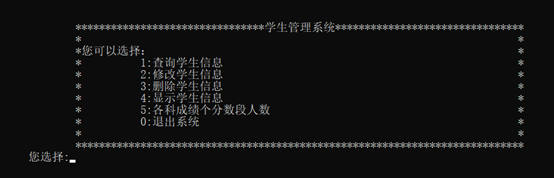2. 查询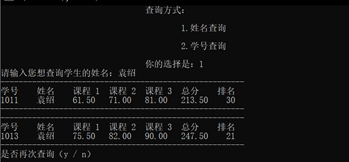3. 修改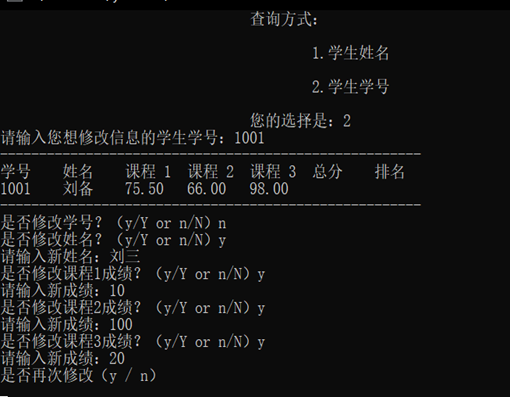4. 删除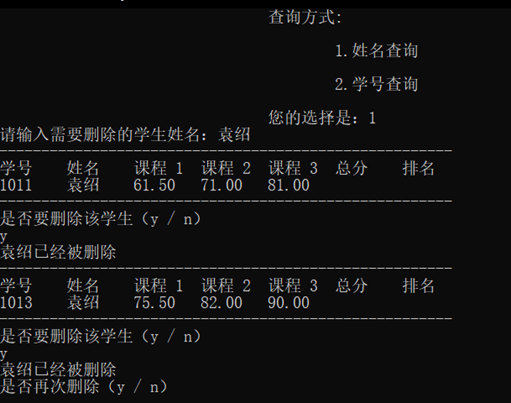5. 显示所有人信息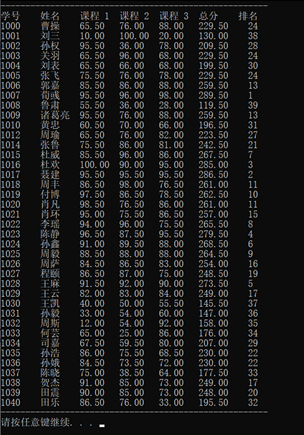6. 各科分数段人数统计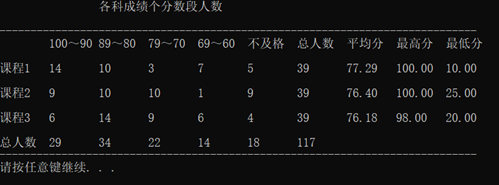7. 退出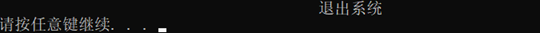8. 数据样式（data1.txt）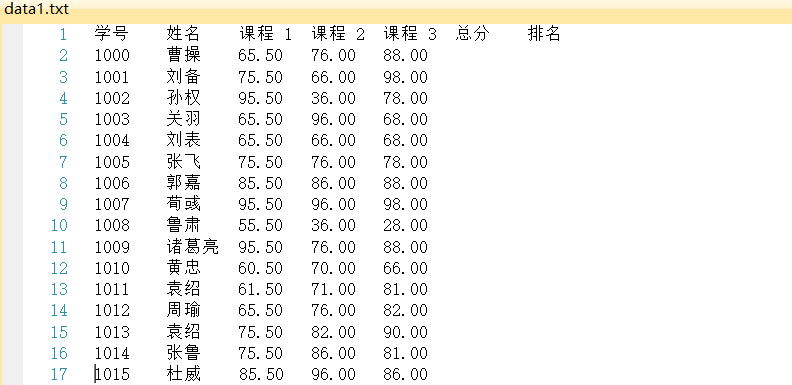9. 数据样式（data2.txt）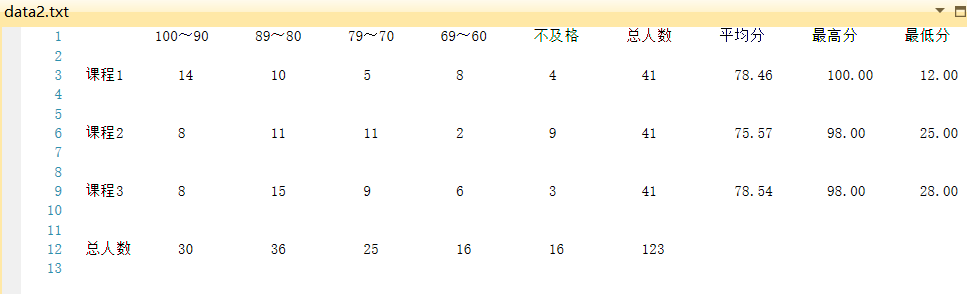1. 数据样式（data3.txt）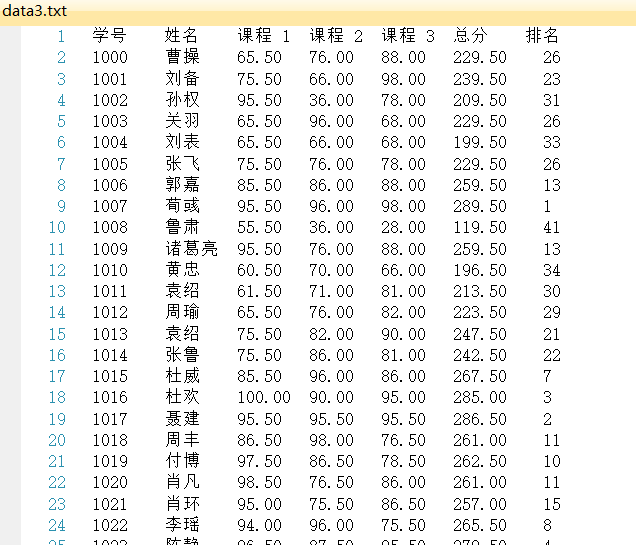展开全文c语言
• C语言进阶 （一）前言： 在学习c语言基础语法后我们需要通过...（二）题目要求：学生成绩统计系统实现 设计结构体数组，结构中包含学生数据为：学号、姓名、物理分数、数学分数、外语分数、计算机分数。 设...

C语言进阶

（四）完整代码实现

# include<stdio.h>
# include<stdlib.h>
# include<string.h>
# include<ctype.h>

struct Student         #自定义结构体
{
int      number;
char     name;
int      phy;
int      math;
int      eng;
int      com;
}stu;

int choose_carte()   #菜单显示
{   int c;
do{ system("cls");
printf("\t\t*********请选择该学生成绩统计系统所实现功能*********\n");
printf("\t\t*1.录入：输入学生数据                              *\n");
printf("\t\t*2.显示：显示学生数据                              *\n");
printf("\t\t*3.统计：统计最高分，最低分                        *\n");
printf("\t\t*0.退出                                            *\n");
printf("\t\t****************************************************\n");
printf("\t\t\tGive you Choice(0-3):");
scanf("%d",&c);
}while(c<0||c>3);
return c;
}

int Intput(struct Student stu[],int n)    #输入学生信息
{
int i=0;
char  c;
do
{
printf("\t\t\t学号：");
scanf("\t\t\t%d",&stu[n+i].number);
printf("\t\t\t姓名：");
scanf("\t\t\t%s",&stu[n+i].name);
printf("\t\t\t物理分数：");
scanf("\t\t\t%d",&stu[n+i].phy);
printf("\t\t\t数学分数：");
scanf("\t\t\t%d",&stu[n+i].math);
printf("\t\t\t外语分数：");
scanf("\t\t\t%d",&stu[n+i].eng);
printf("\t\t\t计算机分数：");
scanf("\t\t\t%d",&stu[n+i].com);
i++;
printf("\t\t\t您还要继续录入成绩吗？\n");
printf("\t\t\t请输入‘y’继续，或'n'停止");
scanf("\t\t\t%c",&c);
}while(c=='y');
return (i+n);
}

int Output(struct Student stu[],int n)       #输出学生信息
{
int i;
printf("\t\t----------------------------------------------------\n");
printf("\t\t| Number |  Name  |  Phy  |  Math |  Eng  |  Com  | \n");
printf("\t\t----------------------------------------------------\n");
for( i=1;i<n+1;i++)
{
printf("\t\t%4d%10s%9d%9d%9d%9d\n",stu[i-1].number,stu[i-1].name,stu[i-1].phy,stu[i-1].math,stu[i-1].eng,stu[i-1].com);
printf("\t\t----------------------------------------------------\n");
}
system("pause");

}

void Data(struct Student stu[],int n) #统计
{
int i;
int sum=0;
int phy_hight=0;
int math_hight=0;
int eng_hight=0;
int com_hight=0;
int phy_low=0;
int math_low=0;
int eng_low=0;
int com_low=0;
for(i=0;i<n;i++)
{
if(stu[phy_hight].phy<stu[i].phy)
phy_hight=i;
if(stu[phy_low].phy>stu[i].phy)
phy_low=i;
if(stu[i].phy<60)
printf("\t\t物理不及格的%4d%10s%9d\n",stu[i].number,stu[i].name,stu[i].phy);

if(stu[math_hight].math<stu[i].math)
math_hight=i;
if(stu[math_low].math>stu[i].math)
math_low=i;
if(stu[i].math<60)
printf("\t\t数学不及格的%4d%10s%9d\n",stu[i].number,stu[i].name,stu[i].math);

if(stu[eng_hight].eng<stu[i].eng)
eng_hight=i;
if(stu[eng_low].eng>stu[i].eng)
eng_low=i;
if(stu[i].eng<60)
printf("\t\t英语不及格的%4d%10s%9d\n",stu[i].number,stu[i].name,stu[i].eng);

if(stu[com_hight].com<stu[i].com)
com_hight=i;
if(stu[com_low].com>stu[i].com)
com_low=i;
if(stu[i].phy<60)
printf("\t\t计算机不及格的%4d%10s%9d\n",stu[i].number,stu[i].name,stu[i].com);
++sum;
}
printf("\t\t该数据中不及格的人数为%d\n",sum);
system("pause");
printf("\t\t物理最高分为:\n");
printf("\t\t%4d%10s%9d\n",stu[phy_hight].number,stu[phy_hight].name,stu[phy_hight].phy);
printf("\t\t数学最高分为:\n");
printf("\t\t%4d%10s%9d\n",stu[math_hight].number,stu[math_hight].name,stu[math_hight].math);
printf("\t\t外语最高分为:\n");
printf("\t\t%4d%10s%9d\n",stu[eng_hight].number,stu[eng_hight].name,stu[eng_hight].eng);
printf("\t\t计算机最高分为:\n");
printf("\t\t%4d%10s%9d",stu[com_hight].number,stu[com_hight].name,stu[com_hight].com);
printf("\n\n\n");

printf("\t\t物理最低分为:\n");
printf("\t\t%4d%10s%9d\n",stu[phy_low].number,stu[phy_low].name,stu[phy_low].phy);
printf("\t\t数学最低分低为:\n");
printf("\t\t%4d%10s%9d\n",stu[math_low].number,stu[math_low].name,stu[math_low].math);
printf("\t\t外语最低分为:\n");
printf("\t\t%4d%10s%9d\n",stu[eng_low].number,stu[eng_low].name,stu[eng_low].eng);
printf("\t\t计算机最低分为:\n");
printf("\t\t%4d%10s%9d\n",stu[com_low].number,stu[com_low].name,stu[com_low].com);
system("pause");

}

void main()
{
int n=0;
do{
switch(choose_carte())
{
case 0:
break;
case 1:
printf("\t\t\t请输入学生的个人信息\n");
n=Intput(stu,n);
break;
case 2:
printf("\t\t\t输出所有学生的个人信息\n");
Output(stu,n);
break;
case 3:
Data(stu,n);
break;
}

}while(choose_carte()!=0);
}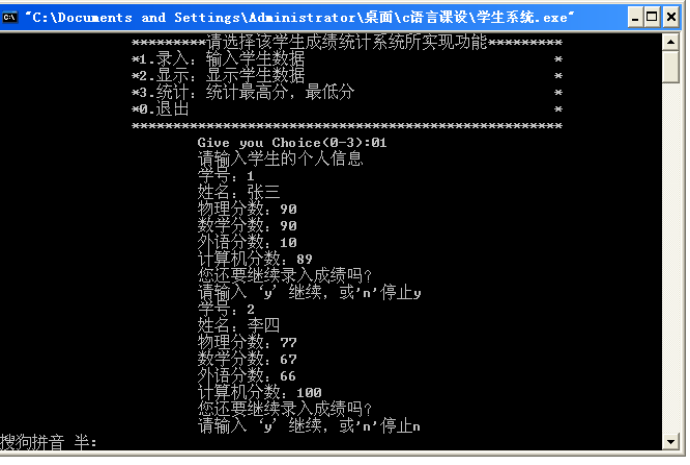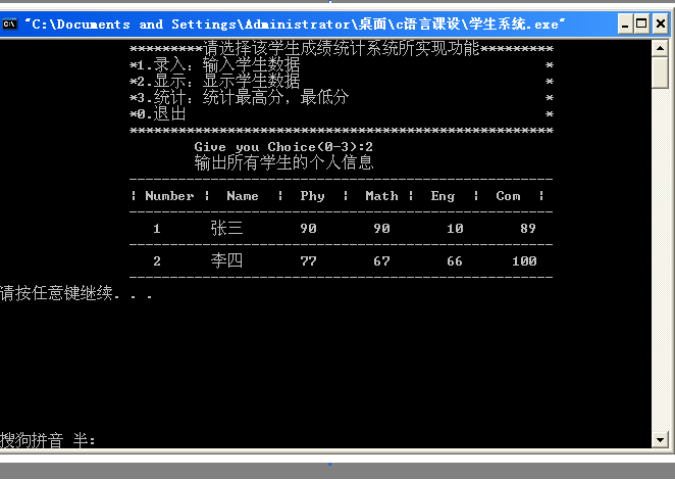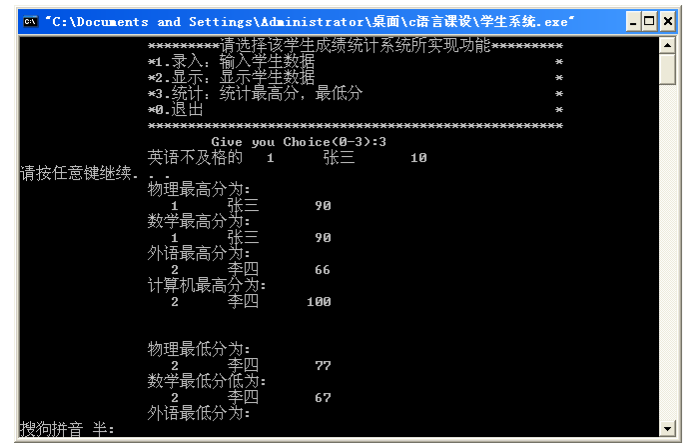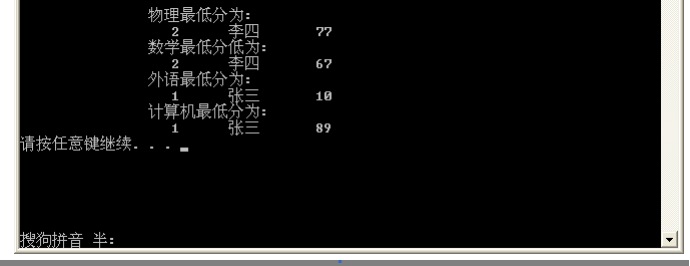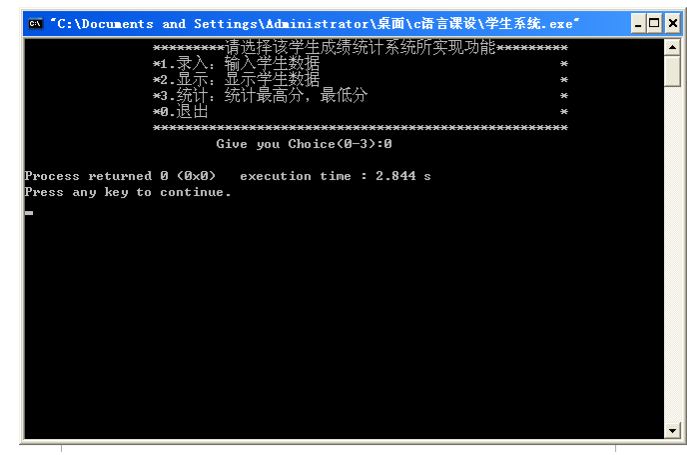（六）项目小结

要熟练掌握循环用来控制显示面板和所要调用的函数，结构清晰有便于后期系统的调试和运行，要使用暂停函数system("pause");让数据停留，综合性非常高，也需要对每个数据非常严谨的编写。

展开全文• C语言开发简单的学生成绩管理系统，包含如下功能： 1.录入学生信息：用户可以自由输入学生信息到系统中。 2.打印学生信息：格式化展示系统中的学生信息。 3.保存学生信息：将系统中的学生信息保存到本地文档。 4....
• 学习C语言后的一次设计与总结，程序设计与问题求解基础...它是一个简单的学生成绩管理信息系统学生成绩信息包括：学号，姓名，课程名，平时成绩，实验成绩，考试成绩，总评成绩，可对信息进行增删查改，排序，评优等
• 课 程 设 计课程名称____C语言课程设计___题目名称____学生成绩统计_____学生学院______物理学院_______专业班级____电子科学与技术___学 号_____3110008570______学生姓名______胡健___________指导教师_______刘...

课 程 设 计

课程名称____C语言课程设计___

题目名称____学生成绩统计_____

学生学院______物理学院_______

专业班级____电子科学与技术___

学 号_____3110008570______

学生姓名______胡健___________

指导教师_______刘力斌________

2012 年 10 月 17 日

C语言课程设计报告

一、C语言课程设计的目的：

进一步消化和巩固已学过的C 语言程序设计基础理论，学习和实践程序设计的各种技能，利用C 语言来设计各种应用程序和管理系统，为社会提供各种现代化的管理工具。根据课堂讲授内容，做相应的自主练习，消化课堂所讲解的内容；通过调试典型例题或习题积累调试C程序的经验；通过完成辅导教材中的编程题，逐渐培养学生的编程能力、用计算机解决实际问题的能力。在许多常规学科的日常教学中，我们不难发现这样一个现象，不少学生的思维常常处于混乱的状态。写起作文来前言不搭后语，解起数学题来步骤混乱，这些都是缺乏思维训练的结果。程序设计是公认的、最能直接有效地训练学生的创新思维，培养分析问题、解决问题能力的学科之一。即使一个简单的程序，从任务分析、确定算法、界面布局、编写代码到调试运行，整个过程学生都需要有条理地构思，这中间有猜测设想、判断推理的抽象思维训练，也有分析问题、解决问题、预测目标等能力的培养。

模式(mode)1：新建一个工程；

模式(mode)2：修改；

模式(mode)3：删除；

模式(mode)4：插入；

模式(mode)5：查看。

Stu.num :学生学号；

Stu.name:学生名字(注：拼音)；

Stu.math:学生数学成绩；

Stu.physics:学生物理成绩；

Stu.english:学生英语成绩；

Stu.clanguage:学生C语言成绩；

Stu.moral:学生德育成绩；

Stu.ave:学生平均成绩；

模式1：将会弹出对话“Stu.num：”，输入学号，回车，以此类推。

模式2：将会询问你需要修改哪一位的数据，按照弹窗提示操作。

模式3：将会询问你需要删除哪一位的数据，按照弹窗提示操作。

模式4：按照弹窗提示操作。

模式5：直接弹出所有学生的成绩，按排名高低输出。

输出文件名：“stu.dat”

【分析】

该程序能够存储学生成绩数据，排序，算出平均值以及删除，修改，插入等功能，具有较强的实用性。

全程序主要模块包括“排序模块”，“不及格学生输出模块”，“文件保存以及输出模块”以及“成绩排名模块”。“排序模块”模块的难点在于结构体数组的赋值是否合法以及字符函数的运用，“成绩排名”的难点在于判断各模式之间的联系。“文件保存以及输出模块”的难点在于文件操作函数的繁杂以及情况的判断，“不及格学生输出模块”的难点在于成绩的判断以及输入输出的排序。

/***************************************************************************

技术文档/帮助清单

***************************************************************************/

这是一个学生成绩统计程序。该程序仅以10人为例，可通过修改宏定义N来指定人数新建程序。在VC++6.0运行。

/************************************************************

模式(mode)1：新建一个工程；

模式(mode)2：修改；

模式(mode)3：删除；

模式(mode)4：插入；

模式(mode)5：查看。

Stu.num :学生学号；

Stu.name:学生名字(注：拼音)；

Stu.math:学生数学成绩；

Stu.physics:学生物理成绩；

Stu.english:学生英语成绩；

Stu.clanguage:学生C语言成绩；

Stu.moral:学生德育成绩；

Stu.ave:学生平均成绩；

************************************************************/

模式1：将会弹出对话“Stu.num：”，输入学号，回车，以此类推。

模式2：将会

展开全文• C 语言课程设计学生成绩管理系统 个人心得体会 徐州工程学院 14 计嵌 1 班 07 组 张凯 这一周以来的课程设计不仅让我明白了 C 语言的确是一门很有用的但是又是不容 易的一门课程 起初刚开始做课程设计时 以为这个...
• C语言：学生成绩统计系统

千次阅读 多人点赞 2016-11-09 22:40:21
该程序可以输入学生成绩，计算平均成绩和及格率，以及查找学生成绩和修改学生成绩，输入所需功能对应的数字再按回车键即可，效果如下 ： 看起来还不错吧，代码如下： #include void main() { int sel,i=0,x,...C语言
• 设计一个学生信息管理系统，包括学生成绩信息数据的求和、排序、查找、模糊查找、分类统计、输出、读写文件等基本操作，要求编程实现如下菜单驱动的学生信息管理系统： （1）录入每个学生的学号、姓名、学院和各科...C语言
• 3.学生成绩统计：计算每个学生的平均分并根据学生的平均成绩高低进行排后输出。 4.学生成绩修改：对已经录入学生成绩信息（学号、姓名、成绩）进行修改。 5.学生成绩保存：对已经录入学生成绩信息进行保存。 6....C语言
• 学生成绩统计分析系统，分为五大模块：信息录入模块、信息查看模块、信息修改模块、信息排序模块、信息统计分析模块。采取全局变量的方式，统筹模块中的函数。学生成绩信息包括学号、姓名、语文成绩、数学成绩、英语...
• 《学生学分管理程序-c语言代码-学生成绩统计c语言代码》由会员分享，可在线阅读，更多相关《学生学分管理程序-c语言代码-学生成绩统计c语言代码(20页珍藏版)》请在人人文库网上搜索。1、程序设计基础实训项目名称：...
• 成绩统计系统c语言.doc成绩统计系统一目的通过课程设计，加深对《C语言程序设计》课程所学知识的理解，熟练掌握和巩固C 语言的基本知识和语法规范，包括：数据类型(整形、实型、字符型、指针、数组、结构 等)；...c语言成绩统计系统
• 记一次课程设计作业学生成绩管理系统#include#include#include#include/*学生成绩管理系统介绍2017C语言课程设计2021609361.支持将数据保存到文件并从文件中读取已有数据2.支持添加、删改数据3.支持按学号或姓名查询...
• C语言 学生成绩统计及学籍管理系统 萌新第一次做的C语言大作业，所做内容与题目要求略有不同，添加了在输入成绩时禁止输入字母和负数的程序，添加了在输入成绩时禁止大于100分的程序，首次使用需要先添加一个保存...c语言
• #define STU_NUM 10 /*宏定义学生的数量*/struct student /*定义一个结构体用来存放学生学号、三门课成绩、总分及平均成绩*/{char stu_id; /*学生学号；*/float score; /*三门课成绩；*/float total; /*总...
• C语言-学生成绩管理系统02--可用来学习C语言 1. 可实现对学生信息进行登记、删除、查询、修改的基本功能 2. 可对录入的信息进行保存，并采用文件存储的形式 3. 可输出全部学生的信息，从文件中读取 4. 可对学生各科...
• C语言小程序-学生成绩统计系统

万次阅读 多人点赞 2019-05-30 13:12:45
C语言小程序-学生成绩统计系统 #刚入门的小白，写的一个C语言作业，参考了 另一位博主的代码(https://blog.csdn.net/qq_36503589/article/details/53106983) 但是我自信的认为我的代码更“好看”一点，见笑了，请...C语言程序
• c语言版的学生成绩管理系统，有ppt和Word文档，分管理员账户与学生账户，代码长度1000行+；是一个完整的实验报告
• 请教c语言高手~~~~ 设计一个学生成绩管理系统 功能： 1.学生成绩的录入.最低0.27元/天开通百度文库会员，可在文库查看完整内容> 原发布者：beibeifc 用C语言实现一个简单的学生成绩管理系统摘要：伴随着现代社会...
• 学生成绩管理系统 开发语言：C语言 开发工具：Visual Studio 2019 开发时间：2019.4.14 开发者：summer @一、系统使用展示 @二、系统功能 @三、菜单 @四、录入学生信息 @五、打印学生信息 @六、保存学生信息 @七、...C语言 链表 学生成绩管理系统
• 移通学院 C 语言程序设计报告书 设计名称学生成绩管理系统 专业 指导老师 班级 姓名 学号 座号 时间 1 一实验目的 1.在编辑应用程序过程中逐步加深对C 语言编程的理解 2.加强实践的能力动手能力同时进一步对所学的...
• 学生成绩管理系统 系统功能模块如下： 1.学生信息管理:实现对学生信息(包括学号、姓名、性别、班级)数据的增删改查操作。 2.班级信息管理:实现对班级信息(包括班级编号、班级名称)数据的增删改查操作。 3.课程信息...c#
• 福建工程学院计算机和信息科学系试验汇报20XX – 20XX 年第 1 学期 任课老师： 章静课程名称结构化程序综合设计班级计算机1001座号姓名张扬文试验题目学生成绩管理系统试验时间试验开始日期：20XX/2/24 汇报提交日期...学生成绩计算的c语言
• 学生成绩管理系统C语言版

千次阅读 多人点赞 2019-08-13 16:52:04C语言
• 设计语言：C语言，实现10个人5门成绩的求和排序，求平均排序，每门成绩排序。...

学生成绩统计系统c语言c语言 订阅